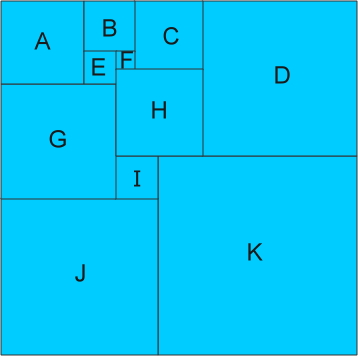## 11個正方形數學謎題A=41，B=25，C=34，D=77，E=16，F=9，
G=57，H=43，I=21，J=78，K=99

#### 解析

bbbnnn(奇風), larry(岸輔 鷓)...等 3 人共同編輯 | 歷史版本

E和F相鄰，因此設E、F為未知數，可依序得到：
B=E+F
C=B+F=E+2F
H=C+F=E+3F

D=C+H=2E+5F
A=B+E=2E+F
G=A+E=3E+F

I=A+G-D=3E-3F
J=G+I=6E-2F
K=I+J=9E-5F

177=A+B+C+D=6E+9F
176=A+G+J=11E

6,609